Documentation

# dsp.HighpassFilter

FIR or IIR highpass filter

## Description

The `dsp.HighpassFilter` System object™ independently filters each channel of the input over time using the given design specifications. You can set the `FilterType` property of `dsp.HighpassFilter` to `'FIR'` or `'IIR'` to implement the object as an FIR or IIR highpass filter.

To filter each channel of your input:

1. Create the `dsp.HighpassFilter` object and set its properties.

2. Call the object with arguments, as if it were a function.

To learn more about how System objects work, see What Are System Objects? (MATLAB).

## Creation

### Syntax

``HPF = dsp.HighpassFilter``
``HPF = dsp.HighpassFilter(Name,Value)``

### Description

````HPF = dsp.HighpassFilter` returns a minimum order FIR highpass filter, `HPF`, with the default filter settings. Calling the object with the default property settings filters the input data with a stopband frequency of `8` kHz, a passband frequency of `12` kHz, a stopband attenuation of `80` dB, and a passband ripple of `0.1` dB.```

example

````HPF = dsp.HighpassFilter(Name,Value)` returns a highpass filter, with additional properties specified by one or more `Name,Value` pair arguments. `Name` is the property name and `Value` is the corresponding value. `Name` must appear inside single quotes (' '). You can specify several name-value pair arguments in any order as `Name1,Value1,...,NameN,ValueN`.```

## Properties

expand all

Unless otherwise indicated, properties are nontunable, which means you cannot change their values after calling the object. Objects lock when you call them, and the `release` function unlocks them.

If a property is tunable, you can change its value at any time.

For more information on changing property values, see System Design in MATLAB Using System Objects (MATLAB).

Input sample rate in Hz, specified as the comma-separated pair consisting of `'SampleRate'` and a positive real scalar.

Data Types: `single` | `double`

Filter type, specified as one of the following options:

• `'FIR'` — The object designs an FIR highpass filter.

• `'IIR'` — The object designs an IIR highpass (biquad) filter.

Minimum order filter design, specified as the comma-separated pair consisting of `'DesignForMinimumOrder'` and a logical value. If this property is `true`, then `dsp.HighpassFilter` designs filters with the minimum order that meets the passband frequency, stopband frequency, passband ripple, and stopband attenuation specifications. Set these specifications using the corresponding properties. If this property is `false`, then the object designs filters with the order that you specify in the `FilterOrder` property. This filter design meets the passband frequency, passband ripple, and stopband attenuation specifications that you set using the respective properties.

Order of the FIR or IIR filter, specified as the comma-separated pair consisting of `'FilterOrder'` and a positive integer scalar.

#### Dependencies

Specifying a filter order is only valid when the value of `'DesignForMinimumOrder'` is `false`.

Data Types: `single` | `double` | `int8` | `int16` | `int32` | `int64` | `uint8` | `uint16` | `uint32` | `uint64`

Filter stopband edge frequency in Hz, specified as the comma-separated pair consisting of `'StopbandFrequency'` and a real positive scalar. The value of the stopband edge frequency in Hz must be less than the passband frequency.

#### Dependencies

You can specify the stopband edge frequency only when `'DesignForMinimumOrder'` is `true`.

Data Types: `single` | `double` | `int8` | `int16` | `int32` | `int64` | `uint8` | `uint16` | `uint32` | `uint64`

Filter passband edge frequency in Hz, specified as the comma-separated pair consisting of `'PassbandFrequency'` and a real positive scalar. The value of the passband edge frequency in Hz must be less than half the `SampleRate` and greater than `StopbandFrequency`.

Data Types: `single` | `double` | `int8` | `int16` | `int32` | `int64` | `uint8` | `uint16` | `uint32` | `uint64`

Minimum attenuation in the stopband in dB, specified as the comma-separated pair consisting of `'StopbandAttenuation'` and a real positive scalar. Minimum attenuation in the stopband defaults to `80` dB.

Data Types: `single` | `double` | `int8` | `int16` | `int32` | `int64` | `uint8` | `uint16` | `uint32` | `uint64`

Maximum ripple of filter response in the passband, in dB, specified as the comma-separated pair consisting of `'PassbandRipple'` and a real positive scalar. Maximum ripple of filter response defaults to `0.1` dB.

Data Types: `single` | `double` | `int8` | `int16` | `int32` | `int64` | `uint8` | `uint16` | `uint32` | `uint64`

#### Fixed-Point Properties

Rounding method for output fixed-point operations, specified as a character vector. For more information on the rounding modes, see Precision and Range.

Word and fraction lengths of coefficients, specified as a `numerictype` object. The default, `numerictype(1,16)` corresponds to a signed numeric type object with 16-bit coefficients and a fraction length determined based on the coefficient values, to give the best possible precision.

This property is not tunable.

Word length of the output is same as the word length of the input. Fraction length of the output is computed such that the entire dynamic range of the output can be represented without overflow. For details on how the fraction length of the output is computed, see Fixed-Point Precision Rules for Avoiding Overflow in FIR Filters.

## Usage

For versions earlier than R2016b, use the `step` function to run the System object algorithm. The arguments to `step` are the object you created, followed by the arguments shown in this section.

For example, `y = step(obj,x)` and `y = obj(x)` perform equivalent operations.

### Syntax

``y = HPF(x)``

### Description

example

````y = HPF(x)` highpass filters the input signal, `x`. `y` is a highpass-filtered version of `x`.```

### Input Arguments

expand all

Noisy data input, specified as a vector or a matrix. If the input signal is a matrix, each column of the matrix is treated as an independent channel. The number of rows in the input signal denote the channel length. This object accepts variable-size inputs. After the object is locked, you can change the size of each input channel, but you cannot change the number of channels.

Data Types: `single` | `double` | `int8` | `int16` | `int32` | `int64` | `uint8` | `uint16` | `uint32` | `uint64` | `fi`
Complex Number Support: Yes

### Output Arguments

expand all

Filtered output, returned as a vector or a matrix. The output has the same size, data type, and complexity characteristics as the input.

Data Types: `single` | `double` | `int8` | `int16` | `int32` | `int64` | `uint8` | `uint16` | `uint32` | `uint64` | `fi`
Complex Number Support: Yes

## Object Functions

To use an object function, specify the System object as the first input argument. For example, to release system resources of a System object named `obj`, use this syntax:

`release(obj)`

expand all

 `freqz` Frequency response of filter `fvtool` Visualize frequency response of DSP filters `impz` Impulse response of discrete-time filter System object `info` Information about filter System object `coeffs` Filter coefficients `cost` Estimate cost for implementing filter System objects `grpdelay` Group delay response of discrete-time filter System object `generatehdl` Generate HDL code for quantized DSP filter (requires Filter Design HDL Coder) `measure` Measure frequency response characteristics of filter System object
 `step` Run System object algorithm `release` Release resources and allow changes to System object property values and input characteristics `reset` Reset internal states of System object

## Examples

expand all

Create a minimum order FIR highpass filter for data sampled at 44.1 kHz. Specify a passband frequency of 12 kHz, a stopband frequency of 8 kHz, a passband ripple of 0.1 dB, and a stopband attenuation of 80 dB.

```Fs = 44.1e3; filtertype = 'FIR'; Fpass = 12e3; Fstop = 8e3; Rp = 0.1; Astop = 80; FIRHPF = dsp.HighpassFilter('SampleRate',Fs,... 'FilterType',filtertype,... 'PassbandFrequency',Fpass,... 'StopbandFrequency',Fstop,... 'PassbandRipple',Rp,... 'StopbandAttenuation',Astop);```

Design a minimum order IIR highpass filter with the same properties as the FIR highpass filter. Use `clone` to create a system object with the same properties as the FIR Highpass filter. Change the `FilterType` property of the cloned filter to `IIR`.

```IIRHPF = clone(FIRHPF); IIRHPF.FilterType = 'IIR';```

Plot the impulse response of the FIR highpass filter. The zeroth order coefficient is delayed by 19 samples, which is equal to the group delay of the filter. The FIR highpass filter is a causal FIR filter

`fvtool(FIRHPF,'Analysis','impulse')`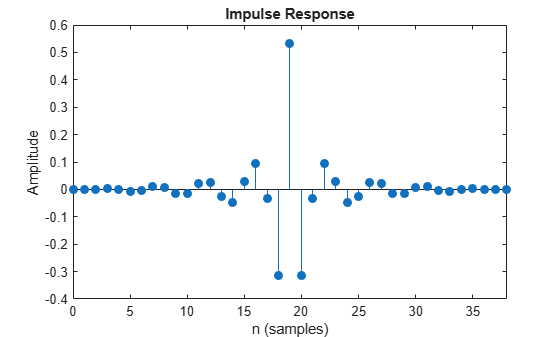Plot the impulse response of the IIR highpass filter.

`fvtool(IIRHPF,'Analysis','impulse')`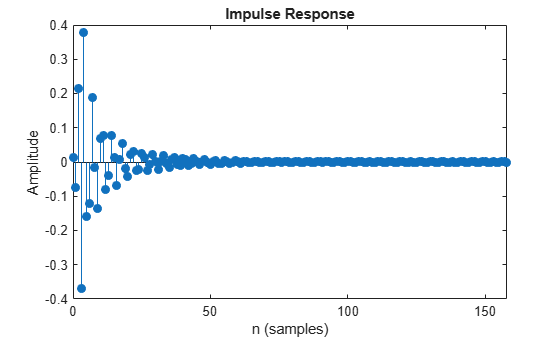Plot the magnitude and phase response of the FIR highpass filter.

`fvtool(FIRHPF,'Analysis','freq')`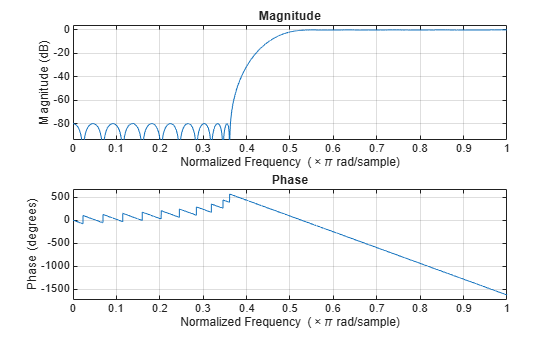Plot the magnitude and phase response of the IIR highpass filter.

`fvtool(IIRHPF,'Analysis','freq')`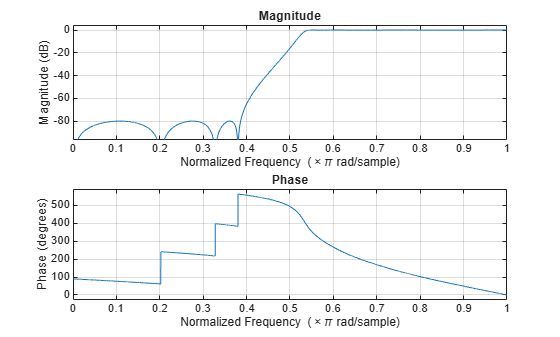Calculate the cost of implementing the FIR highpass filter.

`cost(FIRHPF)`
```ans = struct with fields: NumCoefficients: 39 NumStates: 38 MultiplicationsPerInputSample: 39 AdditionsPerInputSample: 38 ```

Calculate the cost of implementing the IIR highpass filter. The IIR filter is more efficient to implement than its FIR counterpart.

`cost(IIRHPF)`
```ans = struct with fields: NumCoefficients: 18 NumStates: 14 MultiplicationsPerInputSample: 18 AdditionsPerInputSample: 14 ```

Calculate the group delay of the FIR highpass filter.

`grpdelay(FIRHPF)`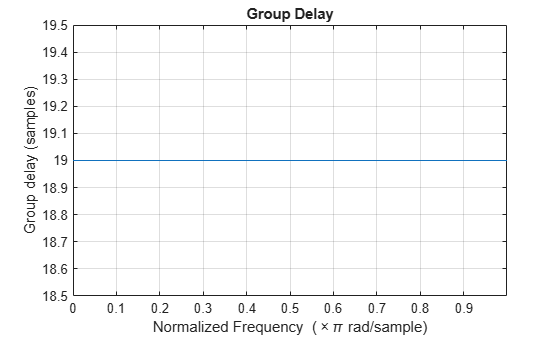Calculate the group delay of the IIR highpass filter. The FIR filter has a constant group delay (linear phase) while its IIR counterpart does not.

`grpdelay(IIRHPF)`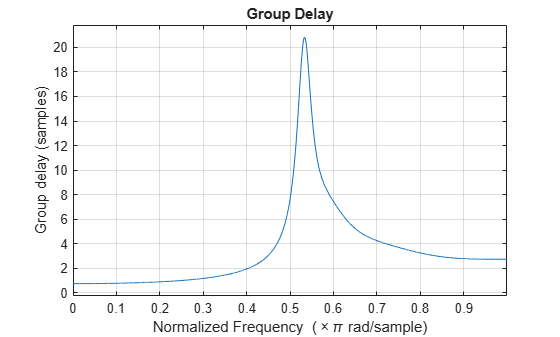Note: This example runs only in R2016b or later. If you are using an earlier release, replace each call to the function with the equivalent `step` syntax. For example, myObject(x) becomes step(myObject,x).

Set up the IIR highpass filter. The sampling rate of the white Gaussian noise is 44,100 Hz. The passband frequency of the filter is 12 kHz, the stopband frequency is 8 kHz, the passband ripple is 0.1 dB, and the stopband attenuation is 80 dB.

```Fs = 44.1e3; filtertype = 'IIR'; Fpass = 12e3; Fstop = 8e3; Rp = 0.1; Astop = 80; hpf = dsp.HighpassFilter('SampleRate',Fs,... 'FilterType',filtertype,... 'PassbandFrequency',Fpass,... 'StopbandFrequency',Fstop,... 'PassbandRipple',Rp,... 'StopbandAttenuation',Astop); ```

View the magnitude response of the highpass filter.

```fvtool(hpf) ```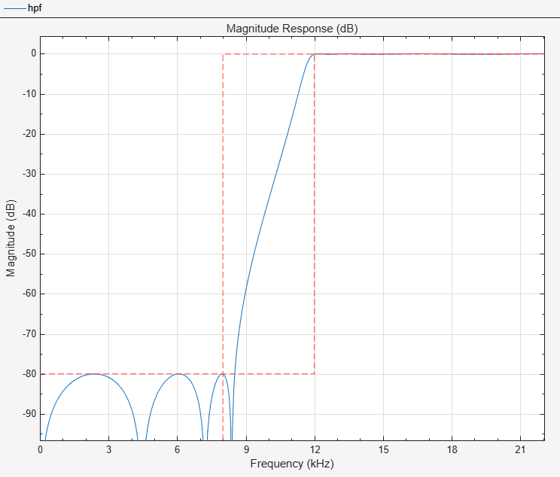Create a spectrum analyzer object.

```sa = dsp.SpectrumAnalyzer('SampleRate',44.1e3,... 'PlotAsTwoSidedSpectrum',false,'ShowLegend',true,'YLimits',... [-150 30],... 'Title',... 'Input Signal and Output Signal of IIR Highpass Filter'); sa.ChannelNames = {'Input','Output'}; ```

Filter the white Gaussian noisy input signal. View the input and output signals using the spectrum analyzer.

```for k = 1:100 Input = randn(1024,1); Output = hpf(Input); sa([Input,Output]); end ```Measure the frequency response characteristics of a highpass filter. Create a `dsp.HighpassFilter` System object with default properties. Measure the frequency response characteristics of the filter.

`HPF = dsp.HighpassFilter`
```HPF = dsp.HighpassFilter with properties: FilterType: 'FIR' DesignForMinimumOrder: true StopbandFrequency: 8000 PassbandFrequency: 12000 StopbandAttenuation: 80 PassbandRipple: 0.1000 SampleRate: 44100 Show all properties ```
`HPFMeas = measure(HPF)`
```HPFMeas = Sample Rate : 44.1 kHz Stopband Edge : 8 kHz 6-dB Point : 10.418 kHz 3-dB Point : 10.8594 kHz Passband Edge : 12 kHz Stopband Atten. : 81.8558 dB Passband Ripple : 0.08066 dB Transition Width : 4 kHz ```

## Algorithms

expand all

 Shpak, D.J., and A. Antoniou. "A generalized Remez method for the design of FIR digital filters." IEEE® Transactions on Circuits and Systems. Vol. 37, Issue 2, Feb. 1990, pp. 161–174.

 Selesnick, I.W., and C. S. Burrus. "Exchange algorithms that complement the Parks-McClellan algorithm for linear-phase FIR filter design." IEEE Transactions on Circuits and Systems. Vol. 44, Issue 2, Feb. 1997, pp. 137–143.

Watch now